5.10. Doppler Effect

5.10.1 General

Doppler effect in electromagnetic phenomena has been often used in order to prove expansion of the Universe . Availability of the model of the photon and the laws of its motion allows us to check correctness of interpretation of infrared and ultraviolet biases of the spectral lines formed by emission of the stars of the Universe.

It is known that the explanation of the bias of the spectral lines in modern physics is based on Doppler effect, which is manifested and registered easily during acoustic wave propagation.

An electromagnetic wave (Fig. 4) is formed by the photon flux. The distance between the pulses of modulated wave is equal to the length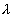of the electromagnetic wave.

It can be easily seen that during electromagnetic wave propagation Doppler effect (Fig. 4) is similar to acoustic wave propagation. The change of electromagnetic emission  (Fig. 4) depends of velocities and directions of motion both of the source and the receiver of such emission. For example, if the directions of motion of the source or the reflector of such wave coincide with the direction of motion of the wave itself, its frequency is increased. Each photon, which forms such wave, acts in a different way.

If the directions of motion of the photon source and the photons themselves (Fig. 5) coincide (Fig. 5), frequency of the photons being born is increased as well, and the spectrum is displaced into ultraviolet range. If in a similar case the photon is not born, but reflected, its wavelength is increased in accordance with Compton effect, frequency is decreased, and the spectrum is displaced into infrared range. It remains unclear if the wavelength and frequency of a separate photon depend on the direction of motion and velocity of its receiver. It becomes necessary to analyse Doppler effect in detail during emission of not an electromagnetic wave, but of separate photons (Fig. 5).

It appears from the analysis of motion of the model of the photon (Fig. 5) that the process of its creation is a transient phenomenon. The essence of Doppler effect is in the fact that the electrons of the source emit the photons with smaller or larger energy. The change of durability of the transient phenomenon stipulated by various directions of the photon emission in relation to the direction of motion of the source is the most probable cause of it.

The longer the photon creation process, which we call the transient phenomenon, the larger mass (energy) will be given to the electron by the photon, and the wavelength of such photon will be displaced into infrared range. That’s why it is necessary to find the answer to a fundamental question: does emission source velocity in relation to space influence durability of the transient phenomenon, i.e. the photon creation process? If so, durability of the transient phenomenon should depend on the direction of the start of the photon in relation to emission source motion direction. As the transient phenomenon takes place actually in the electromagnetic field of the electron of the emission source, there is every reason to believe that the photon mass can be changed during this transient phenomenon.

It appears from the analysis of kinematics of the  photon model motion (Figs 5, 6) that the increase of its velocity from any initial value to value C always takes place with acceleration, which is generated by the process of interaction between its electromagnetic fields. That’s why we should get mathematical models describing to the first approximation  the process of the start of the photon from a resting source and a moving source, due to which the spectra are displaced , , . There are two interpretations of this effect: the relativistic interpretation and the classical one , , .

5.10.2. The Relativistic Interpretation of the Doppler Effect

The relativistic interpretation is based on the second Einstein’s postulate: Any ray of light moves in the stationary system of co-ordinates with the determined velocity, whether the ray be emitted by a stationary or a moving body. The Lorentz transformations originate from this wording of the postulate (3) and (4).

It is clear from Fig. 2  that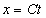and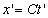. If we insert these values in the formula (1) or (2), we’ll find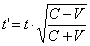(113)

or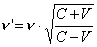,                                                                                 (114)

where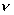and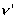are frequencies of electromagnetic emission in the moving and stationary reference systems,  respectively.

If we designate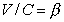,  we’ll have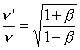(115)

This is the relativistic mathematical model of the calculation of the Doppler effect. As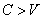, it results from the ratios (114) that emission frequencyof the moving source is greater than radiation frequency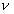of stationary source, i.e. the mathematical models (114) and (115) describe only ultraviolet shift of the spectra.

If we write the ratio (115) in the following form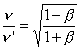,                                                                                   (116)

it will show to what extent frequencyof the photon emitted from the stationary source (Fig. 2) is less than frequency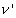of the photon emitted by the moving source, and it will not characterize the infrared shift of the spectra. As in both formulas (114), (115) and (116)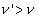, both formulas describe the ultraviolet shift of the spectra, and we have no right to use the mathematical model (116) for the calculation of the infrared shift of the spectra.

Let us prescribe several values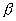and determine values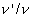and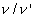for them (Table 2).

## Table 2. Relativistic results of calculation of the Doppler effect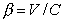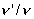(115)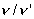(116) 0.000001 0.00001 0.0001 0.001 0.01 0.1 1.0000009 1.0000099 1.0000999 1.0010004 1.0100504 1.10554 0.9999989 0.9999899 0.9998999 0.9990004 0.9900494 0.904534

The results of  Table 1 show unambiguously that frequencyof the emitted photon is increased with the increase of velocity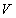of movement of the reference system (for example, of a star). It means that an ultraviolet shift of the spectral lines is increased. Physical sense being present in mathematical symbols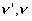anddeprives us of the right to give another interpretation of the mathematical models (114),  (115) and (116).

Thus, we have got an unambiguous answer: the relativistic mathematical models (115) and (116) describe only ultraviolet shift of the spectra, and they are not connected with their infrared shift.

In science, the Lorentz transformations (3) and (4) have been used for the calculation of the so called relativistic effects for about a hundred years. Now we get to know that they give the possibility to calculate the relativistic effects only for the ultraviolet shift of the spectra and provide no information concerning the relativistic effects by the infrared shift of the spectra. It means that the Lorentz transformations misrepresent the reality.

We’ll not repeat Lorentz transformation analysis, which proves disagreement of these conversion to the space-matter-time unity axiom, but we’ll note that Doppler effect analysis result being obtained gives us the right to call Lorentz transformations a theoretical virus.

It results from the above mentioned facts that within nearly a hundred years the Lorentz transformations play the role of a theoretical virus in exact sciences, and the scientific community cannot get rid of it. Now we have a powerful means to struggle against this virus: the space-matter-time unity axiom. That’s why there is a hope for convalescence of scientific cognition.

# 5.10.3. Classical Interpretation of the Doppler Effect

The photon creation process analysis carried out by us ,  has shown that it is a transient phenomenon, during which the photon (Fig. 5) moves with acceleration. It appears from this that the transient phenomenon duration depends on the direction of the emitter and the nascent photon , . It gives us the reason to adjust the wording of the second Einstein’s postulate and to formulate it in the following way: Velocity of the photons emitted by a stationary source or a moving one is constant in relation to space and does not depend on the traffic direction of the source and the value of its velocity .

Thus, velocity of the photons is constant in relation to space. The photon creation is a transient phenomenon, during which it moves with acceleration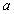and achieves velocity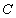in relation to space and moves further with the same velocity , .

If the source is stationary (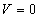), the photon creation process will be put down in the following way (Fig. 9)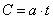.                                                                              (117)

From (117), we have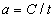.                                                                              (118)

The photon creation process analysis has shown that this process takes place in the wavelength interval , that’s why when the source is stationary  (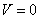), frequencyof emitted photon will be equal.                                                                         (119)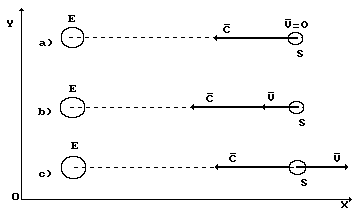Fig. 9. Diagram of addition of velocities of source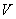and photon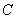:  E is an observer, S is a source

When the directions of movement of the source and the nascent photon (Fig. 9, b) coincide, it means that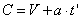,                                                                         (120)

where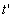is time, during which the photon moves with acceleration.

If we insert acceleration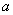from (118), we’ll find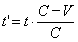.                                                                          (121)

An important corollary originates from the mathematical model (121): if the direction of movement of the emitter and the nascent photon coincide (Fig. 9, b), timeof transient process is reduced with the increase of traverse velocity.

If we proceed to frequencies of the emitted photon, we have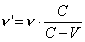.                                                                      (122)

As, it means that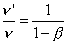.                                                                             (123)

The mathematical models (122)  shows the increase of frequency of the photon when the directions of its velocity C and velocity of the source V coincide (Fig. 9, b). Thus, when the directions of velocities of the source and the nascent photon coincide, an ultraviolet shift of the spectra is observed.

If the directions of the source and the nascent photon are opposite (Fig. 9, c), it means that  frequencyof the photon being emitted is reduced, and the infrared displacement of the spectra should be observed.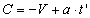.                                                                      (124)

If we take into consideration the ratio (118), we’ll have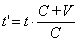;                                                                          (125)

It appears from this that if the direction of movement of the source and the nascent photon  are opposite (Fig. 9, c),  timeof transient process is increased with the increase of traverse velocity. If we proceed to frequencies, we’ll have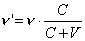(126)

It results from formula (126) that if the directions of movement of the source and the nascent photon are opposite (Fig. 9, c), frequencyof the emitted photon is reduced, and the infrared shift of the spectra can be observed.

The independence of the shift of the spectra from the direction and velocity of movement of the observer is the main conclusion from the analysis of the classical mathematical models (122) and (126).

Taking into account thatwe’ll find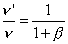.                                                                           (127)

Now let us write the results of the calculation according to the formulas (115), (123) and (127) in Table 3.

Table 3. Results of calculation of the Doppler Effect(123)(127)(115) 0.000001 0.00001 0.0001 0.001 0.01 0.1 1.000001 1.000010 1.000100 1.001000 1.010000 1.100000 0.999999 0.999990 0.999900 0.999000 0.990000 0.900000 1.0000009 1.0000099 1.0000999 1.0010004 1.0100504 1.1055400

The analysis of Table 3 shows that the classical mathematical model (123) describes ultraviolet shift of the spectra when, and the classical mathematical model (127) describes infrared one (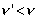). The relativistic mathematical model (115) describes only ultraviolet shift of the spectra  (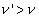).

Now we have an opportunity to explain Doppler effect, which is registered during photon emission, by various durability of transient process of photon creation.

The process of separation of the photon from the electron is not a simultaneous one. The bond between them is preserved for some time. Photon mass, which it takes with it separating from the electron, depends of duration of preservation of this bond. It is clear from the ratio (120) that if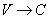,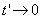. It means that the start of the photon in the direction of motion of a source, which moves in relation to space with velocity, is impossible. In this case, no photon will be emitted by the electron.

When the direction of motion of the emitted photon coincides with the direction of motion of the source (Fig. 9, b), duration (121) of the transient process is reduced as compared with the duration of the transient process at the start from the resting source. Wavelength and frequency of such photon are shifted to the ultraviolet range of the spectrum.

When the photon starts in the direction opposite to the motion of the source (s. Fig. 9, c), duration of the transient process is increased as it is clear from ratio (125), and we have every reason to suppose that in this case in the process of loss of the bond with the electron the photon will give more electromagnetic mass and will arrive to receiver E with the wavelength and frequency shifted to the infrared range.

The same phenomenon takes place when space rockets are launched. When a rocket starts to the east, its velocity coincides with the Earth rotation direction, and it will require less time and less fuel for insertion as compared with the start with the direction to the west.

When the direction of velocities of the source and the photon coincide, duration of the transient process (121) is smaller; if there is no coincidence, duration is larger (125) when the photon emission source is at rest . In the first case (Fig. 9, b), the photon loses less energy (mass) at its creation, and it will arrive to us with energy shifted into the ultraviolet range; in the second case (Fig. 9, c) it loses more mass, and it will arrive to the receiver with smaller energy (mass) shifted into the infrared range.

A question arises: how does the photon give a part of its electromagnetic mass to the electromagnetic field of the electron of the source? The north poles and the south ones of the fields of the photon alternate on its external contour. If the photon in the moment of its creation or reflection by one of its magnetic fields interacts with similar field of the electron emitting it, a bond will be established via the magnetic lines of force at the opposite polarities between these fields.

Thus, the electron of the atom of the emission source with its field will try to hold the photon with the help of magnetic lines of force, via which mass of electromagnetic field (to be more exact, the field itself) of the photon will flow to the electron of the atom of the emission source. The slower the photon withdraws, the greater mass will be lost by it. It seems that other particles are characterized by this process of energy transfer as well. As during this process “mass” is pumped quasi from one particle into another having no opportunity to become a photon of energy (s. Fig. 5), this part of energy is not registered in the experiment .

The revealed corpuscular nature of the photon gives every reason to return to a ballistic hypothesis based on Newton’s concepts concerning light as a flow of material corpuscles , . But this hypothesis acquires a considerable limitation. Here is its essence.

If a stationary reference system is connected with space vacuum and if motion of the source emitting the photons is considered in this system, velocity of emitted photons in relation to the reference system chosen in such a way will be one and the same all the time and equal todespite of the direction of motion and emission source velocity. This result is stipulated by the fact that persistence of photon motion velocity is generated by the electromagnetic processes, which take place in its electromagnetic structure.

Figuratively, the essence of the photon emission process can be compared with the shots from a gun of such shells that despite of initial velocity, with which they leave the barrel, could gain one and the same velocity in relation to the stationary reference system connected with space. The peculiarity of the photon ballistic hypothesis originates from this fact: the lack of the phenomenon of the Galilean addition of velocities of the source and the photon being emitted. When the photon is emitted, it always gains one and the same velocity itself in relation to space, which is equal to. But the Galilean addition of velocities is preserved completely when the photon meets the receiver, but it exerts no influence on energy state of the photon itself.

Certainly, the formulas (123) and (127) are purely kinematics ones, that’s why they express the kinematics process of photon emission approximately. As the electrodynamics of the photon emission process has not been worked out yet, let us use the mathematical models, which describe energy indices of the photons. In this case, the details of the process of their emission remain concealed, but the main index (frequency of the emitted photon) is calculated more precisely than during the use of the

Kinematics mathematical models (123) and (127).

As it is clear, in Table 3 the contradictions between the calculations according to a classical formula (123) and a relativistic formula (115) are increased with the increase of. There exist the experimental evidences that within the increasethe relativistic formula (115) expresses reality more exactly . As the relativists think, it serves as a solid argument of authenticity of the Special Theory of Relativity. Let us give a classical formula, which gives the same result, but it calculates not only the ultra-violet shift of the spectra, but the infrared one as well.

We have already shown that total energy of the photon is equal to the sum of energies of its translational motion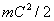and rotational motion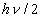and that this amount depends on the value of speedand direction of radiation source motion. If the angle between the direction of velocity vector of source motionand the direction of velocity vector of the photon being emitted (Fig. 10) is equal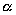, total energy of the emitted photon will be written in the following way .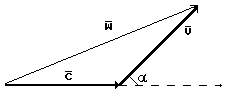Fig. 10. Diagram of addition of velocities of the source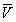and the photon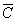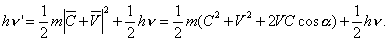(128)

If we take into account that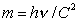and designate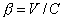, we’ll find after conversion of the equation (128)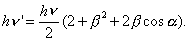(129)

If the directions of the motion of the source and the photon being emitted coincide, it means that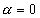and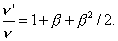(130)

When the directions of the motion of the source and the photon being emitted are counter, it means that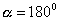and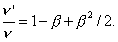(131)

In Table 2, the calculation results are given according to the classical formulas (130) and (131) and relativistic formulas (115) and (116). Analysis of this table shows that the classical formula (130) gives the result, which is close to the result of the relativistic formula (115), and classical formula (131) gives the result, for which there is no relativistic formula.

Relativists use the formula (116) for the calculation of the infrared shift of the spectra having no mathematical right for it. Such right and such accuracy are given by the classical mathematical formula (131) (Table 4).

Table 4. Calculation results of Doppler Effect(130)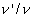(131)(115)(116) 0.000001 0.00001 0.0001 0.001 0.01 0.1 1.0000010 1.0000100 1.0001000 1.0010000 1.0100500 1.1050000 0.9999990 0.9999900 0.9999000 0.9990005 0.9900500 0.9050000 1.0000009 1.0000099 1.0000999 1.0010004 1.0100504 1.1055400 0.9999989 0.9999899 0.9998999 0.9990004 0.9900494 0.9045340

The results of the simultaneous registration of the usual spectral lines of the hydrogen atom received from the space object SS433 and the spectral lines shifted to the ultraviolet and infrared field of the spectrum can serve as a classical experimental fact, which confirms equity of mathematical models (130) and (131). It proves the fact that the main part of the space object SS433 is stationary in relation to space, and two other parts move in relation to space. The part, which generates the ultraviolet shift, moves to the Earth, and the part, which generates the infrared shift at that time, moves from the Earth. Periodicity of the change of values of these shifts is registered , , .

# 5.11. Is the Universe Expanded?

At present, the infrared shift of the spectral lines formed by the starts of the galaxies is the main evidence of expansion of the Universe. The question concerning the influence of direction and velocity of the emission receiver on the value of this shift remains undetermined.

Thus, the photon start process does not influence on its final velocity in relation to space, and its duration (117), (120) depends on the direction of motion of the emission source and the photon in relation to space.

The given analysis of Doppler Effect taking into consideration the photon model (Fig. 5) shows independence of any shift of the spectral lines from the direction of motion and velocity of emission receiver. The value and the direction of the shift (into infrared or ultraviolet range of the spectrum) depend on the direction of motion of the emission source and the emission itself. If these directions coincide, only the ultraviolet shift of the spectral lines should be observed; if these directions are opposite, only the infrared shift is observed. This regularity shows that availability of the infrared shift of the spectral lines is not enough for unambiguous conclusion concerning the expansion of the Universe.

As the Earth moves in relation to space, it should be taken into account during the analysis of the connection of the shift of the spectral lines with the expansion of the Universe.

For example, if the vectors of  velocities of the Earth and the star are directed along one line in one and the same side, the value of the shift of a spectral line will point to the fact of motion of the star in relation to space, but not in relation to the Earth. In this case if the Earth follows the star with velocity in relation to space greater than velocity of the star, these celestial bodies will approach each other. But due to the fact that the time of the start of the photon from the star in direction to the Earth is increased (125) (as compared with), we’ll register the infrared shift of the spectral lines (126). It means that the distance between the star and the Earth is reduced at the infrared shift of the spectra.

If the star follows the Earth with greater velocity than the Earth, in this case the celestial bodies will approach each other, but the time of the start (121) of the photon in the direction to the Earth will be less than at, and we’ll register the ultraviolet shift (122). Thus, in both cases the star and the Earth approached each other, but the shift of the spectral lines were opposite.

Can the motion of the star in relation to the Earth influence on the shift of the spectral lines? Certainly, not. This process is governed by velocity of the star not in relation to the planets or galaxies, but in relation to one thing, which is common for all stars, planets and galaxies: in relation to space.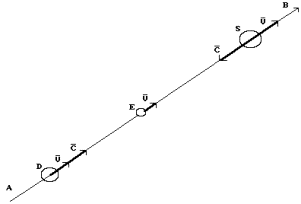Fig. 11. Diagram to the analysis of expansion of the Universe: AB is a radial direction of the expansion of the Universe; D, E and S are the galaxies situated on the radial direction of expansion of the Universe; E is our galaxy.

The important result of the analysis of the spectra of the source SS433 is the fact that the ultraviolet shift of the spectral lines is 20fold less than the infrared one by equal velocities of motion . Obviously, due to this fact the astrophysicists register mainly the infrared shift of the spectral lines of the majority of stars, and on this basis they make a conclusion concerning expansion of the Universe. Availability of ultraviolet shift of the spectra with some stars denotes that the infrared shift of the spectral lines is an insufficient condition for an unambiguous conclusion concerning expansion of the Universe. This conclusion will be unambiguous only in case when both infrared and ultraviolet shifts of the spectra are taken into consideration.

In order to make an unambiguous conclusion concerning expansion of the Universe, it is necessary to register a shift of the spectra from the opposite points of the surface of the Earth (Fig. 11).

If the infrared shift is registered in one direction (for example, from source S) and the ultraviolet shift is registered in the opposite direction (from source D), the conclusion concerning expansion of the Universe can be accepted as the unambiguous one. If this regularity is not confirmed, the infrared shift of the spectra is not connected with the expansion of the Universe; a cause of this shift is different, and we should find it.

5.12. Brief Conclusion

Lorentz transformation analysis carried out with the help of the space-matter-time unity axiom shows clearly and conclusively that in exact sciences they play the role of a theoretical virus.

The relativistic mathematical model (114), which originates from the Lorentz transformations, has nothing to do with the Doppler effect.

The classical wording of the second Einstein’s postulate opens the new possibilities in the solution of the fundamental tasks of physics .

The ultraviolet and infrared shifts of the spectra describe the classical mathematical models (122) and (126), which originate from the classical wording of the second Einstein’s postulate. They will give more exact results when their connection with the mathematical model of the formation of the spectra of the atoms and the ions is established.

The Doppler Effect of electromagnetic wave (Fig. 4) depends on the direction of the motion of the receiver and its velocity.

The Doppler Effect of the separate photon (Fig. 5) does not depend on the direction of the motion of the receiver and its velocity.

The modern conclusion concerning expansion of the Universe made only on the basis of the analysis of the infrared shift of the spectra cannot be accepted as an unambiguous one.

We have considered the simplest cases of Doppler Effect when the emission source of the electromagnetic wave or single photon moves to the receiver or from it. The electromagnetic wave (Fig. 4) and single photons (Fig. 5) behave similarly in both cases.

The cases of interaction of electromagnetic wave and the single photons with the receiver remain unconsidered. But the structure of the electromagnetic wave (Fig. 4) gives the reason to suppose that velocity and direction of motion of the receiver of such a wave will generate Doppler Effect as well. The photon model structure (Fig. 5) points to the fact that is parameters do not depend on velocity and direction of motion of the receiver of the single photons.

If we consider the process of reflection of the electromagnetic wave, velocity and direction of motion of the reflector will generate the same Doppler Effect as velocity and direction of motion of the source.

Single photons forming the electromagnetic wave will behave differently  when they meet the reflector, because the reflector performs two functions: the function of the receiver of single photons and the function of their reflection. In this case, Compton Effect will govern the shift of the spectral lines of the photons. That’s why the process of the change of the parameters of the single photons in interaction with the moving reflector should be carried out taking Compton Effect into consideration.

The main result of Doppler Effect is Galilean addition of velocities of the photons and their receivers.

The Foundations of Physchemistry of Microworld

Ó2003 Kanarev Ph. M.

Internet Version - http://book.physchemistry.innoplaza.net

<< Back to Physchemistry Book Index

 This appropriateness is concealed in the transient process of nascence of the photon, the detailed analysis of which waits for its investigator.